# Program Scalability Analysis

Yuan Shi

Computer and Information Sciences Department

Temple University (MS:38-24)

shi@falcon.cis.temple.edu

215/204-6437(Voice) 215/204-5082(Fax)

April 1996

# ABSTRACT

Analyzing a parallel algorithm means predicting the resources that the algorithm requires, such as time, memory, disk space and communication bandwidth. For most parallel programs time is of the primary concern. Generally, by analyzing many candidate algorithms for solving a given problem, a most efficient one can often be identified. Such an analysis may indicate more than one viable candidates, but several inferior ones are often discarded in the process. In this paper we propose a methodology for analyzing parallel programs. In particular, this method can assess the scalability of sequential and parallel programs.

Analyzing the scalability of a algorithm means predicting its potential elapsed times for varying input size, processor speed, communication network and file system speeds. The results can be useful in the design, implementation and maintenance of practical applications.

We use a complexity-based method to capture the performance critical parameters and their impact on the overall system elapsed time. The sequential program's elapsed time is the basis of comparison. Therefore scalability analysis requires models for both the sequential and the parallel algorithms. We call these timing models.

Timing models capture the essential performance critical parameters in one equation. Scalability analysis begins with a calibration of the base points, i.e., uni-processor speed, file system and network speeds. We then can scale up/down any parameters to conduct what-if analysis.

This paper introduces techniques in building and using steady state timing models via a set of diverse algorithms. Computational results are used to validate the timing model predictions.

Keywords: Parallel programming, performance evaluation, scalability analysis, parallel program analysis.

## 1. Introduction

Analyzing a parallel algorithm means predicting the resources that the algorithm requires, such as time, memory, disk space and communication bandwidth. For most parallel programs, we assume time is of the primary concern. Generally, by analyzing many candidate algorithms for solving a given problem, a most efficient one can often be identified. Such an analysis may indicate more than one viable candidates, but several inferior ones are often discarded in the process.

Unlike conventional program complexity analysis, program scalability analysis requires two specifications: a) characteristics of an algorithm ; and b) characteristics of the processing environment. For each program, a timing model should be established using these specifications in order to generate estimated elapsed times. Scalability analysis is to investigate the changes in elapsed times while we alter algorithmic and processing specifications.

In this paper, since our main interests are in complexity analysis, we use the words algorithm and program interchangeably.

### 1.1 Sequential Program Scalability Analysis

In sequential program scalability analysis, computing and input/output are the two major timing factors. For example, a typical sort program requires O(nlgn) computing steps and O(n) bytes for input/output when processing an input of size n. This gives a simple timing model as follows:

T =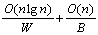(1.1)

where W is the computing speed in "instructions per second" and B is the file system's speed in "bytes per second". We further assume that each input consists of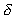bytes and W is a customized measure in "number of inputs per second". This way the constant in the time complexity O(nlgn) can be "hidden" in W. Thus, the new model becomes:

Tseq=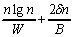(1.2)

To begin scalability analysis, we need to establish the base points of the program performance map by some computational experiments. In this example, we first measure B by timing the read and write blocks in the sort program that transfersbytes 2n times. We then run the sort program to obtain the total elapsed time (Tseq) sorting a problem of size n. Finally, we derive a customized W using B, n,and (1.2). These numbers establish the base point of the performance map for the sort program.

We then use (1.2) to examine the scalability of the sort program by generating performance maps with varying W, B and n. Table 1-3 illustrate the scalability analysis results for the sort program.

 N CPU(sec.) I/O (sec.) Time(sec.) 10000 0.03 0.06 * 0.09 * 20000 0.05 0.13 0.18 40000 0.12 0.26 0.37 80000 0.25 0.51 0.76 160000 0.53 1.02 1.56 320000 1.13 2.05 3.17 640000 2.37 4.10 6.47 1280000 4.99 8.19 13.19 2560000 10.48 16.38 26.86 5120000 21.94 32.77 54.71 10240000 45.86 65.54 111.39 20480000 95.66 131.07 226.73 40960000 199.19 262.14 461.33 81920000 414.13 524.29 938.42 1.64E+08 859.77 1,048.58 1,908.35

* Calibrated points for B=2.5 MB/Sec. and W=5.2 MIPS on a DEC Alpha/120 MHZ processor and NFS mount file system via Ethernet.
Table 1. Sequential MergeSort Scalability by Input Size

 N\B 2.50E+06 3.00E+06 5.00E+06 1.00E+07 2e+07 10000 0.09 0.08 0.06 0.04 0.03 20000 0.18 0.16 0.12 0.09 0.07 40000 0.37 0.33 0.25 0.18 0.15 80000 0.76 0.68 0.51 0.38 0.31 160000 1.56 1.39 1.04 0.79 0.66 320000 3.17 2.83 2.15 1.64 1.38 640000 6.47 5.79 4.42 3.40 2.89 1280000 13.19 11.82 9.09 7.04 6.02 2560000 26.86 24.13 18.67 14.58 12.53 5120000 54.71 49.25 38.33 30.14 26.04 10240000 111.39 100.47 78.63 62.24 54.05 20480000 226.73 204.88 161.19 128.42 112.04 40960000 461.33 417.64 330.26 264.73 231.96 81920000 938.42 851.04 676.28 545.20 479.67 1.64E+08 1,908.35 1,733.59 1,384.06 1,121.92 990.84

Table 2. Scalability by Input Sizes and I/O Speeds (W=5.2MIPS)

 N\W 5.20E+06 1.00E+07 2.00E+07 5.00E+07 10000 0.09 0.08 0.07 0.07 20000 0.18 0.16 0.14 0.13 40000 0.37 0.32 0.29 0.27 80000 0.76 0.64 0.58 0.54 160000 1.56 1.30 1.16 1.08 320000 3.17 2.63 2.34 2.17 640000 6.47 5.33 4.71 4.34 1280000 13.19 10.79 9.49 8.71 2560000 26.86 21.83 19.11 17.47 5120000 54.71 44.18 38.47 35.05 10240000 111.39 89.38 77.46 70.31 20480000 226.73 180.81 155.94 141.02 40960000 461.33 365.72 313.93 282.86 81920000 938.42 739.64 631.96 567.36 1.64E+08 1,908.35 1,495.66 1,272.12 1,137.99

Table 3. Scalability by Input Size and Processor Speeds (B=2.5 MBPS)

From Table 1, we know that MergeSort is an I/O intense program. Tables 2 and 3 illustrate that upgrade to a faster file system can improve the overall processing time more than upgrading the processor.

Note that the processing parameters W and B may not scale linearly due to swapping and other factors. However, modern processing and input/output hardware devices are fast enough that these imprecision can be either tolerated or compensated using simple statistical techniques. Note also that the computational experiments are only necessary if one needs the best possible prediction. Empirical data can often be used if only qualitative measures are needed.

### 1.2 Parallel Program Scalability Analysis

Unlike a sequential program, a parallel program is associated with greater development expenses and illusive potential. The key problem is that there are more performance sensitive parameters in parallel processing than that in sequential programming. However, using simple extensions to the above scalability analysis method, we can establish a parallel program scalability analysis method called steady state timing models.

#### Background

In traditional parallel program evaluation, Amdahl's law  has been most widely used: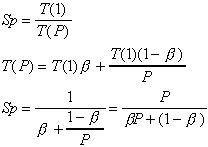where Sp is speedup, T(1) is the execution time using one processor, T(P) is the execution time using P processors,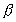is the percentage of total serial part of the program. Thus () of the program can be processed in parallel using P processors.

A cursory reading of Amdahl's Law may result in an assumption thatis a constant for a given serial program. This assumption is incorrect. Gustafson  first recognized that the percentage of total serial partis a function of the number of processors P. Gustafson's formulation attempts to calibrate the sequential execution time T(1) based on the parallel execution time T(P). This is shown as follows: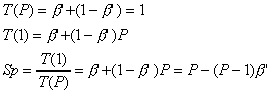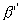is a ratio between the sequential portion elapsed time and the total elapsed time using P processors. The erroneous assumption ofequates this percentage to a ratio between perceived pure sequential portion elapsed time and the total elapsed time using a single processor. The error is that the perceived parallel portion can only be defined when P is known.

To see the differences, let P=10, a parallel execution results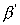=0.6, namely 40% of the elapsed time is spent on parallel processing (using 10 processors) and 60% is for sequential processing. If we considered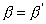, the Amdahl's law would predict Sp = 10/(6.4) =1.6 while Gustafson's law gives Sp = 10 - 5.4 = 4.6.

This difference disappears if we computeaccording to the correct assumption. For example, let 10 seconds be the total elapsed time for the parallel algorithm that gives themeasure. The total sequential elapsed time for the same algorithm should be 46 = 4 10 + 6 seconds. This yields= 6/46 = 0.13. Then the Amdahl's law gives the identical result: Sp = 10/(1.3+0.87) = 4.6.

However, regardless of their equivalence, both laws are too simple to be useful in parallel program scalability analysis. There are three considered deficiencies:

1. The absence of communication costs. This encourages excessively fine grain designs.
2. The empirical nature by the use ofor. Many practical programs contain variable degrees of parallelism that cannot be precisely modeled using simple percentages.
3. The lack of resource capacity specifications. This makes it impossible to assess the overhead caused by the disparities among computing, communication and input/output capacities.

The importance of scalability analysis has long been recognized. In 1980, Ruby Lee defined a model of efficiency, utilization and quality for parallel programs . This model intends to grasp the quality of a parallel program as a ratio of efficiency speedup and redundancy (extent of parallel workload increase). This definition ignores the possibility of non-search space compact algorithms (see Section 2) that can require less parallel workload than their sequential counter-parts. The model also lacked processor and communication capacity specifications.

Harmonic mean speedup  considers a parallel computer with n processors executing m programs in various modes with different performance levels. The harmonic mean performance of such multimode computer is a measure using a weighted distribution. The use of the weighted distribution gives too much latitude that permits one to "bend" the analysis results (towards either in favor of using more processors or in favor of using fewer processors).

Scalability metric  concerns with a list of attributes that are considered important to the scalability of a parallel computer system. It can help to guide parallel program development. However, it lacks a generic analysis tool.

Scalability definition  was based on the ratio of the asymptotic speedup of an algorithm on a real machine to the asymptotic speedup on an ideal realization on an EREW PRAM (Exclusive Read Exclusive Write Parallel Random Access Machine). It does not include any resource specifications, such as processor and network capacities. However, carefully applying this method had found that communication latency is inversely related to scalability .

The LogP model  tracks the communication overhead by analyzing detailed message passing patterns and latencies. It has been used to demonstrate the negative effects by the use of massively many processors . It does not include processing time modeling.

Trace driven simulation systems use computer generated trace information from running a parallel program to track the inter-dependencies of all overheads. It has been useful to identify architecture dependent bottlenecks. However, the cost of such an approach is prohibitively high. The lack of a generic evaluation method makes them useful only for restricted purposes.

According to , the evolution path of scalable computers includes the following measures:

1. Size Scalability -- The study of hardware system scalability with an expectation of linearly increased performance with incremental expansion for a well-defined set of applications.
2. Generation (Time) Scalability -- The measure of compatibility of hardware and (system) software with regard to the passage of time. The intent is to gauge the proportional scaling of all hardware and software components using the passage of time as a reference.
3. Problem Scalability -- The measure of speedup of a computer as the problem size increases.

These measures are influenced by many hard-to-control factors ranging from program decomposition method, compiler efficiency to hardware features.

Given a running parallel program, the key performance contributing parameters of a parallel processing environment are: P (number of processors), W (processing capacity of every processor), B (disk I/O rate), and u (network capacity). Similar to sequential program scalability analysis, we treat these as customized parameters that can be significantly different from manufacturer's specifications. W includes all effects of advanced multiple functional unit, memory and cache support under the influence of the quality of a compiler. B includes disk caching and possible parallel access support, such as provided by the RAID (Redundant Array of Inexpensive Disks) systems. u includes hardware protocol and messaging support effects. It denotes the worst-case latency when all processors are transmitting. For example, if u=10,000 bytes per second, we expect the network to transmit 10,000 bytes in 1 second and 0.5 second for 5,000 bytes even if all processors are transmitting at the same time. It reflects the worst-case deliverable bandwidth and latency.

Similarly, the parallel program can be characterized by: size of the problem (n) it solves, computing density (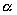), communication density (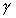) and disk I/O data density () per input. Since these are time independent parameters and can be estimated or measured easily in the serial program.

A steady state speedup model is a ratio between steady state timing models for sequential and parallel algorithms solving the same problem. Since the timing models project all effects into the time domain, the speedup model captures the global interdependencies of all parameters in one equation. An efficiency model can then be obtained (Eff = Sp/P). If the models are precise, the analysis accuracy is only affected by the values of parameters.

The speedup and efficiency models are used to generate performance maps for the given parallel program (and the serial program). These maps reveal speedup and efficiency behavior of the program under any combinations of algorithmic and processing parameters.

Generally, it is hard to develop an abstraction for a parallel program running on multiple parallel processors because there are an explosive number of possibilities with a wide variety of structures. However, from the view point of quantifying the intrinsic dependencies among the key performance contributing parameters of a running parallel program, we can relate all parameters in the time domain by introducing resource capacity specifications as we have done for the sequential program scalability analysis.

### 1.5 Outline of This Paper

In this paper, we use five examples to illustrate the application of steady state timing models. They disclose some counter-intuitive results of well-known algorithms.

• Section 2: Preliminaries.
• Section 3. Sorting in Parallel. It may be tempting to split a large sorting job onto multiple supercomputers connected via high speed links, but timing model results can make you think twice. However, a small change in the sort kernel complexity can result in superlinear speedup using a cluster of workstations via a standard Ethernet.
• Section 4: MPP Limitations. MPP stands for Massively Parallel Processing. This case study quantifies the power increase and efficiency loss when adding processors for an embarrassingly parallel program. Given the problem size and a target efficiency, one can find the best processor/network configuration for this "best-case" MPP application.
• Section 5: Comparing Parallel Programs. This section illustrates how two parallel programs can be meaningfully compared using steady state timing models.
• Section 6: Modeling Exclusive and Broadcast Messages. Matrix multiplication requires both exclusive and broadcast messages when processed in parallel. This example illustrates how to find speedup "bumps" with steady state performance maps.
• Section 7. Modeling Fine Grain and Decreasing Parallelism. We use a direct linear solver to illustrate how to quantify fine grain and decreasing parallelism with complex data sharing patterns.
• Section 8. Finding the Best Grain Size. Parallel processing grain size directly impacts the communication and synchronization overheads. We show that given any serial algorithm, the best parallel processing grain size corresponds to the coarsest level abstraction that preserves the highest level of SIMD, MIMD and pipeline realizations of the serial algorithm. For example, 2-D tiled partitioning is inferior than 1-D striped partitioning.
• Section 9. Accuracy Control. Accuracy control is useful when it is necessary to predict the speedup of a parallel program in a specific parallel processing environment. This section illustrates practical steps for determining accurate algorithmic and processing parameter values.
• Section 10: Conclusions.

Sequential and parallel program examples in this paper have been compiled and run using a cluster of workstations and the Synergy parallel programming system. Example programs and performance data can be retrieved via: .

## 2. Preliminaries and Limitations

In steady state timing analysis we are looking for the lower bound (best-case) parallel execution time (assuming the best load balancing), if the algorithm is solution space compact (see Section 2.1). Otherwise the timing prediction will be the upperbound (worst case) elapsed time. The results are expected to be correct within a constant error in the context of all assumptions.

Throughout this paper, we use the following notations:

```
n : Input size,

P : Number of processors,

Sp : Speedup, and

Eff : Efficiency = Sp/P.

```

We define a base algorithm as a sequential algorithm solving a problem of interest. A correct parallel implementation of the base algorithm should generate identical outputs as the serial algorithm for all possible inputs. In general, there exists at least one correct parallel implementation for every serial algorithm. Conversely, there exists at least one correct serial equivalent (base) algorithm for every possible parallel algorithm.

We base our parallel implementations on the three forms of parallelism related to those of Flynn and others .

```
SI(P)MD : Single Instruction (or Program) Multiple Data

MI(P)MD : Multiple Instruction (or Program) Multiple Data, and

Pipeline : Sequentially dependent multiple processors processing

multiple consecutive data sets.

```

In this paper, SIMD and SPMD, MIMD and MPMD are synonyms. Note that this treatment is different from hardware classifications that consider SPMD as MIMD running multiple copies of the same program.

### 2.1 Superlinear Speedup

We define that speedup is a ratio between a base serial (not necessary the best) program's execution time and its parallel implementation's execution time, e.g. Sp = Tseq/Tpar. There are three possibilities:

• Sp < P, or sublinear speedup;
• Sp = P, or linear speedup;
• Sp > P, or superlinear speedup.

Considering the inherent overheads in parallel processing, we identify two factors for linear and superlinear speedups:

• Use of a resource constrained execution as the comparison base; and
• Reduced total parallel execution steps comparing to the sequential counter-part.

It is relatively easy to create a large program and report a linear or superlinear speedup while the runs using single and smaller number of processors suffer from insufficient resources, such as memory or cache. We consider this an "unfair" practice. In order to identify parallel algorithmic advantages, we prefer "fair" speedup bases -- sequential runs without resource constraints.

Note that an "unfair" speedup is in fact an advantage of parallel processing in that the partitioned parallel programs are typically much less demanding on local resources than their serial counterpart.

Definition 1. A sequential algorithm is search space compact (SSC) if all possible parallel implementations of the same algorithm must require equal or more total number of calculation steps (including those in parallel) for all inputs.

Definition 2. A sequential algorithm is non-search space compact (NSSC) if there exists at least one parallel implementation of the same algorithm, computing at least one input, that requires less total number of calculation steps (including those in parallel) than the total pure sequential steps.

Note that the emphasis of implementing the same serial algorithm in parallel restricts the freedom of parallel implementation only to realizing application dependent SIMD, MIMD and pipelining parallelisms. This restriction applies to both serial to parallel and parallel to serial program transformations.

Definition 3. A certificate is a verification algorithm that given a solution to a problem it can verify if the solution is correct or not.

Theorem 1. Let the complexity of a certificate be f(n) and solution algorithm g(n), the solution algorithm is NSSC if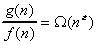, for all n>0 and some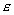> 0.

Example: An O(n2) comparison-based sort algorithm is non-solution space compact, since the sort verification algorithm requires O(n), we find= 1.

In particular, suppose that a serial algorithm requires n2 comparisons to produce a sorted output. Then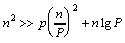, for all n > P >2. (2.1)

In (2.1), the left-hand-side represents the worst-case number of comparisons of the serial sort algorithm, right-hand-side represents the total worst-case parallel computing steps are:

• There are n steps to split the input to P sorters but no comparisons are needed.
• There are P processors each doing (n/P)2 comparisons (SIMD parallelism).
• There are nlgP comparisons to merge the sorted sequences.

There is an apparent reduction in parallel comparison steps! This implies the possible superlinear speedup in parallel execution.

This "magical power" of instruction reduction comes from the way we define our speedup. The multiplicative power of nonlinear functions is the source of "parallel instruction reduction". Such reduction exists in all serial algorithms that are amenable to a divide-and-conquer strategy using exclusively partitioned inputs. Further, for every such NSSC algorithm, there exists an optimal number of partitions of input such that the total number of computing steps is minimized.

On the other hand, an O(nlgn) sort algorithm is SSC since we cannot find an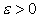.

Corollary 1. All NP-Complete algorithms are non-solution space compact.

Proof:

One condition for an algorithm to be in the NP-complete class is that there exists a certificate that given the solution it can verify the solution in polynomial time . All NP-complete algorithms require exponential time complexity. The conclusion follows.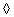Matrix multiplication algorithms are SSC. NP-hard optimization algorithms are SSC. However, for algorithms using branch-and-bound heuristics, the sub-problems are NSSC. Therefore, it is possible for these algorithms to achieve superlinear speedup on selected inputs.

The SSC and NSSC classifications are also applicable to parallel algorithms designed only for parallel machines, such as the line drawing algorithms (SSC) for the massive parallel processor CM2  and the parallel sort algorithms  (SSC).

Note that the NSSC's "parallel instruction reduction power" can be eliminated if we use a "strictly fair" comparison base. For example, for parallel sort, computing speedup using a serial algorithm with the same partitioning factor (a serial divide-and-conquer algorithm) as the number of parallel processors can eliminate superlinear speedup (see Section 3). For optimization algorithms, superlinear speedup can be eliminated if we force the sequential algorithm to following the best parallel search path.

However, in practice, it is inconvenient to alter a serial algorithm whenever we add a processor (for the parallel sort ) or change an input (for optimization algorithms) for performance evaluation. In this paper, we prefer speedup be computed using a "fair" sequential run. Theoretically, superlinear speedup is then only possible for NSSC algorithms. For SSC algorithms delivering superlinear speedups, we know immediately that there is a resource factor in the comparison basis.

### 2.2 Steady State Timing Models

The conventional program time complexity analysis investigates the asymptotic order of execution steps of a program as a function of its input size. The steady state timing model of a program characterizes its asymptotic execution time as a function of performance critical parameters in addition to the input size. In this paper, we use the following:

```
P : Number of processors.

W : Customized processor power (in number of inputs per second).

u : Network capacity (in bytes per second).

B : Disk speed (in bytes per second).

n : Size of problem input.: Computing density (in instructions per input).: Communication density (in bytes per input).: Disk access density (in bytes per input).

```

For convenience, we also use MIPS (Million Inputs Per Second), KBPS (Kilo-Bytes Per Second) and MBPS (Million Bytes Per Second) as aggregate measures.

For a sequential program, the timing model is merely an equation in the following form: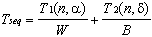. (2.2)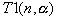is the total number of instructions required to solve a problem of size n.denotes the average calculation steps per input. Different input can changewithout altering the problem size (n). For example, complex domains (-2, -2) (2,2) and (-0.1,-0.1) (0.1,0.1) for a Mandelbrot set displayed on the same screen size can result in drastically different total execution times (Section 8). For other algorithms,can be a constant function implying that the total calculation steps remain the same for all inputs. Matrix multiplication is an example (Section 5).

Similarly,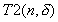is the total disk access volume of the program. If not dominating, we omit T2 in subsequent discussions.

For the parallel program solving the same problem, the timing model contains four basic parts: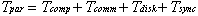(2.3)

where Tcomp is for pure parallel calculation (this corresponds to the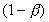part in Amdahl's law), Tcomm is for pure communication, Tdisk is for disk access (possibly in parallel) and Tsync is for program-to-program synchronization. A similar formulation for parallel programs can be found in .

Tsync accumulates all possible waiting times due to serial dependencies. There are two kinds of waiting time:

1. Static -- This refers to the inherent serial computing and communication costs of an application. This loosely corresponds to thepart in Amdahl's law.
2. Dynamic -- This type of synchronization can be minimized at runtime. For example, an scatter-and-gather master can only terminate when all its scattered work assignments are processed. The optimization is to minimize the master's waiting. This is also called "load balancing" in the literature.

In this paper, the dynamic waiting time is set to zero to provide an upper bound for evaluating various load balance schemes.

Having the sequential and parallel timing models, we can easily obtain the speedup and efficiency models as follows: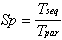, (2.4)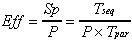. (2.5)

Parallel algorithm scalability analysis is to investigate the performance map generated by values of Sp and Eff under varying algorithmic and processing parameters. Note that for NSSC algorithms we may have Sp >> P and Eff >> 1.

## 3. Sorting in Parallel

Suppose that we are to internally sort a large data set. We have access to a few supercomputers linked by high speed networks and to a few workstations linked by a slow network. We ask if it is worth splitting the data via the network to multiple processors and then merge the results in each of these environments?

### 3.1 An O(nlgn) Sorter Using Supercomputers

Let us use randomized Quicksort . Assuming balanced partitioning and= 8 bytes (size of the sort key), the sequential timing model is: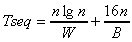. (3.1)

The first term is the pure computing time. The second term is the summation of disk read and write times.

Using the split-sort-merge scheme, we then have a parallel sorting model: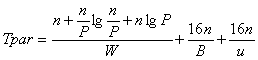. (3.2)

The first term is the computing costs for splitting, parallel sort and merging. The second term is disk I/O. The third term is the total communication cost.

Table 1 is produced using (3.1) and (3.2) with assumptions representing a networked supercomputer system. It illustrates the fact that larger input size has little impact on improving speedup under current assumptions. In this table all measurement units are in seconds, except for n and Sp.

 Time in Seconds Sequential Times Parallel Times n Tcomp_s Tdisk_s Seq. Total Tcomp_p Tdisk_p Tcomm_p Par. Total Sp 1E+09 2.99E+02 1.60E+03 1.90E+03 1.34E+02 1.60E+03 1.60E+02 1.89E+03 1.00 1E+12 3.99E+05 1.60E+06 2.00E+06 1.68E+05 1.60E+06 1.60E+05 1.93E+06 1.04 1E+15 4.98E+08 1.60E+09 2.10E+09 2.01E+08 1.60E+09 1.60E+08 1.96E+09 1.07 1E+18 5.98E+11 1.60E+12 2.20E+12 2.34E+11 1.60E+12 1.60E+11 1.99E+12 1.10 1E+20 6.64E+13 1.60E+14 2.26E+14 2.56E+13 1.60E+14 1.60E+13 2.02E+14 1.12

Assumptions for calculation:

Uni-processor power: W = 100 MIPS

Network capacity (ATM): u = 100 MBPS

Disk I/O capacity (SCSI 2): B = 10 MBPS

Number of processors: P = 3

Table 4. Visualizing Overheads for Sequential and Parallel Sorts

Table 4 also illustrates an important utility of the timing models: visualization of the quantitative overhead changes. In this case, the apparent impeding friction is disk I/O.

In order to find the best parallel processor/network configuration for this application, Figure 2 shows the performance map of the parallel sorter using three processors with varying uni-processor power and networking speeds (P=3, n = 1018 and = 8).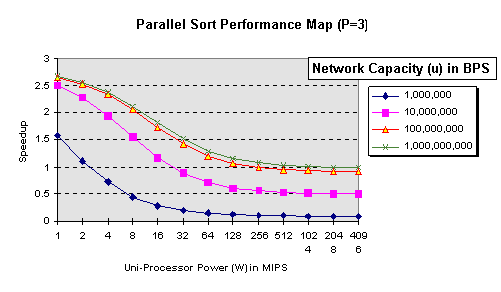Figure 2. Parallel Sort Speedup Map (varying W and u)

Figure 2 illustrates that good speedup is promised if connecting low power processors (<= 1 MIPS) on a fast network (>=100 MBPS). For any network speed, parallel sort using multiple networked fast processors (W > 128 MIPS), a slow disk (10 MBPS) and an O(nlgn) algorithm is not worthwhile.

We can also use these results to investigate possible improvements. For example, we can reduce disk I/O time by changing the second term of (3.2) from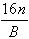to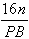. Since P is small, we quickly realize that this will not affect the overall conclusion. However, if the disk system is significantly faster (say 100 times B), this conclusion will change (see next section).

### 3.2 An O(n2) Sorter Using A Cluster of Workstations

Since speedup is a ratio between sequential and parallel running times, inefficient serial programs can result in better speedups (smaller W's). In this section we show how to demonstrate superlinear speedup using a cluster of workstations and an O(n2) sorter.

The sequential and parallel models are as follows: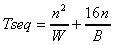, (3.4)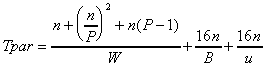. (3.5)

Table 2 shows the predicted (using (3.4) and (3.5)) and achieved speedups using a non-dedicated cluster of 2-5 DEC Alpha workstations. In this environment, uni-processor power W=5MIPS, network capacity (a low-end Ethernet) u=60,000 BPS and disk I/O capacity B=3.5 MBPS (NFS mounted via Ethernet).

 SpeedUp Problem Size (n) (Sp) 1,000 10,000 100,000 P Projected Actual Projected Actual Projected Actual 2 0.64 0.1371 2.60 2.7918 3.79 4.6267 3 0.70 0.21 4.06 4.01 8.02 9.42 4 0.72 0.293 5.05 5.0995 13.15 17.5114 5 0.73 0.34 5.70 5.94 18.67 21.73

Table 5. Predicted and Recorded Superlinear Speedups

For this environment, larger problem sizes caused better speedups. The lower uni-processor power (5 MIPS) has caused the higher computing/IO and computing/ communication ratios.

As mentioned earlier, superlinear speedup would be impossible if we insisted on the best serial program as the comparison basis. For example, partitioning the input in the serial program should improve the sequential execution performance without using parallel processors. Table 3 shows the elapsed times (in seconds) by dividing and solving the problem in 2 and 4 subsets using one processor. This explains why any O(n2) sort algorithm is NSSC (non-search space compact).

 Elapsed t (Sec.) Problem Size (n) Partition: 1,000 10,000 100,000 None 0.25 21.00 2,607.00 2 0.16 10.64 1,091.00 4 0.15 5.04 557.00

Table 6. Elapsed Times of Sequential Sorter Using Partitions

Finally, as suspected, in this processing environment a single workstation using an O(nlgn) algorithm can beat the best multi-processor run using O(n2) algorithms.

## 4. MPP Limitations

MPP stands for Massively Parallel Processing. It denotes the use of many fine grained parallel processors. One often wonders if a given program can effectively take advantage of all these processors. We use an embarrassingly parallel example to quantify the efficiency loss when using many processors even with little communication overhead and very high computing density.

This example involves plotting a fractal image of (x,y) resolution. This requires calculating the color indices of n = x y pixels. The color of each pixel represents the orbit length of a corresponding complex point based on a simple equation (such as Fatou's). Since each pixel can be calculated entirely independent of all others, we can use as many processors as pixels. Further, parallelism exists at many grain levels -- we have at least three ways to split the calculations: by pixel, by rows or columns, and by tiles. Many graphics, image processing and numerical programs share the same characteristics as this example.

We investigate the following: Is it possible to achieve linear speedup (Sp = P) for a "smart" parallel implementation? Can this program benefit from massively many processors?

In this case, given a complex domain,refers to the average computing steps required per pixel,refers to the average number of data bytes (both in and out) per pixel. Assuming that the local memory latency is negligible, the model is: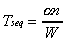. (4.1)

This is an obvious SSC algorithm. Therefore, linear speedup is only possible if we use "unfair" bases.

In the parallel timing model, assuming 1 < P n, the pure computing time is: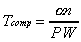.

Since there is no cross data sharing, the total fractal communication time is: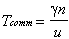.

Assuming further that all processors can terminate at the same time, dynamic synchronization time is zero. No static synchronization exists; thus Tsync = 0. The parallel execution time is: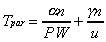. (4.2)

Speedup can be decided: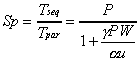. (4.3)

The efficiency is: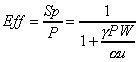. (4.4)

From (4.3) and (4.4), we can conclude the following:

• Unless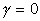, linear speedup is impossible. But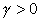for all meaningful parallel implementations. This confirms the SSC characteristics.
• The problem size (n) is not a factor in speedup and efficiency. Contrary to the "folk wisdom" that the larger the problem size causes the better the speedup, this algorithm does not follow the suite.
• The ultimate speedup: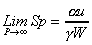. This tells that the weaker the individual processor power (or worse the compiler and operating system, since W is a customized measure), the better the speedup.
• Given an input (by n and) and a parallel processing environment (by u and W), the coarser the parallel grain (larger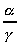ratio) the better speedup and efficiency.

In order to find a desirable aggregate processor power, Figure 3 shows the processor powers using different uni-processors and inter-connection network speeds (varying W and u) for fractal computing. In this calculation, we assumed P=512, computing density=32,000 (instructions per pixel), communication density=8 (number of I/O bytes per pixel).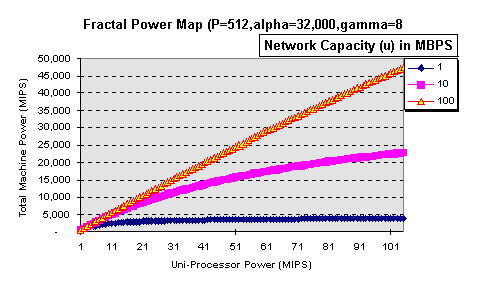Figure 3. Aggregate Processor Powers For Parallel Fractal Computing

Figure 3 suggests that as long as we have fast interconnection network, MPP is a way to acquire larger computing powers. However, the efficiency map suggests something else (Figure 4).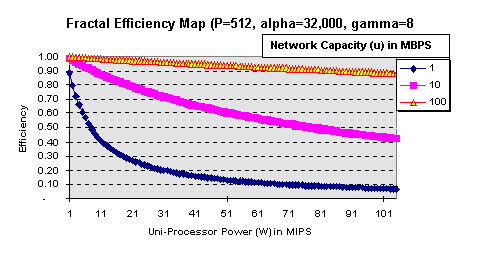Figure 4. Fractal Efficiency Map Using Different Processors and Networks

An 87% efficiency level can be obtained if we use 512 101 MIPS processors with a sustained 100 MBPS network. The use of higher power uni-processors or the use of greater than 512 processors must generate lower efficiency. Also note that these numbers change as the input () changes. This result also implies unless a parallel program is only to process one particular input, it should not rely on a fixed number of processors (logical or physical).

Furthermore, improving theratio is critical to good performance. Sinceis determined by a given input, wiring one processor to each pixel is detrimental to parallel performance (due to largersee Section 9 for details). This is an argument for coarse-grain parallel processing and against fine grain partitioning techniques. This argument also challenges the use of MPP since it is impossible to make efficient use of massively many processors (> 512) even for this "best-case" application.

The "mainstream" parallel processing culture has been on the fine-grain side  even though communication overhead analysis showed adverse effects using too many processors  and . Using the same interconnection network technology, we can build more efficient parallel processors using a fewer high power uni-processors than using massively many weaker processors. It seems that as the uni-processor power increases, there is a clear need to re-evaluate fine grain parallel processing techniques.

## 5. Comparing Parallel Programs

It is usually hard to compare parallel programs. Using steady state timing models, parallel programs can be related by their performance behaviors.

A Monte Carlo simulator typically uses a simulated time (t) that is absent in the previously defined notations. In this case, the size of the problem (n) is the number of units being simulated, e.g., financial instruments, molecules, bodies, etc. In this section, we assume a "typical" Monte Carlo simulator in that for each simulated time slice, the unit computations are independent and data sharing mutually exclusive. Dependencies exist between time slices.

The sequential timing model for this Monte Carlo simulator, without disk I/O, is: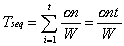. (5.1)

In this case,refers to the average number of computing steps per simulated object per time slice andrefers to the average number of bytes required for input and output per object per time slice.

The parallel timing model, 1 < P n, can be expressed as: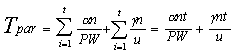. (5.2)

Note that (5.2) includes Tsync in the two summations.

The speedup and efficiency are:. (5.3). (5.4)

Note that (5.3) and (5.4) are identical to (4.3) and (4.4). Therefore, a Monte Carlo simulator should have same performance behavior as a fractal program that is much simpler in structure.

Performance comparisons can help to detect design and coding mistakes. For example, when designing computational experiments, we should notice that both the number of simulated objects and the length of simulated time should have no impact on the speedup and efficiency (unless one wants to manufacture good performance reports with unjustified comparison bases).

## 6. Modeling Exclusive and Broadcast Messages

Matrix multiplication is frequently used in many science and engineering programs. Its common sequential algorithm is as follows. Let A, B and C be n*n matrices and C is initialized to zero:

```
1. for i=1 to n

2.	for j=1 to n

3.		for k=1 to n

4. 			C[i,j] = C[i,j] + A[i,k] * B[k,j]

5. 		end

6. 	end

7. end

```

Line 4 is also called the "dot product" that can be performed independently for each entry in C. This is an SSC algorithm as discussed earlier.

The sequential timing model is: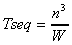(6.1)

For parallel implementations, there are two types of messages: exclusively read and cross shared. Each dot product must use one row of A and one column of B. To prevent doing redundant work, one of them (let's say A) must be mutually exclusively distributed, such that no two processors would get the same row. This amounts to n2 exclusively readable data items on the network. The other matrix (B) must be broadcast to all processors. This requires at most Pn2 data items on the network (assuming no hardware broadcast support). For networks with hardware broadcast support, this may be reduced to O(n2). Using steady state timing models, instead of calculating message delays, we need the total communication volume. This simplifies the modeling process.

Assuming double precision arithmetic (8 bytes per number), the parallel timing model is then: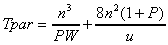(6.2)

The speedup model is: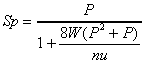(6.3)

This is one of those earliest programs with well documented parallel performance results. (6.3) can be used to validate all published matrix multiplication performance results.

Figure 5 shows the typical speedup map of this system without hardware broadcast support: speedup deteriorates quickly as P increases. This is an example of algorithms with high order computing complexity (n3) and lower order communication complexity (n2). Therefore larger problem size (n) can compensate for the communication costs. The benefit of using steady state models is that we can visualize the order changes and predict the speedup "bumps" prior to programming.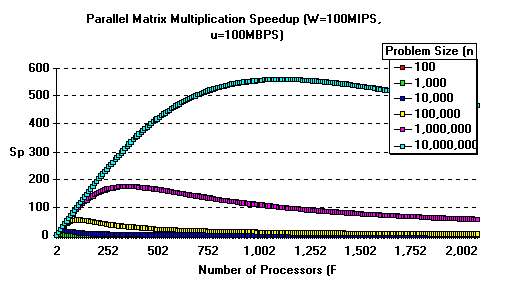Figure 5. Parallel Matrix Multiplication Speedup Prediction

## 7. Modeling Fine-Grain and Decreasing Parallelism

Algorithms for solving linear or non-linear equation systems require large number of serially dependent steps. The key characteristics of this application group are fine grain, dynamic parallelism and frequent communication.

For a linear system Ax=b, Gaussian elimination can be used to find the solutions directly or a factorization of A, such that A=LU where L is a lower-triangular matrix and U is an upper-triangular matrix . Once A is factorized, we can quickly find solutions for different constraints (b's).

Gaussian elimination involves eliminating unknowns one column at a time until we obtain an none-zero upper-triangular matrix (and a lower-triangular matrix of multipliers for LU factorization). We consider the elimination of a column a wave of calculation. There are (n-1) waves in total with the first wave of size (n-1). The second wave must not proceed until the corresponding unknowns are solved in the first wave. The overall triangularization algorithm is to process waves of sizes: (n-1), (n-2), ..., 1.

The variable elimination at each column can be processed in parallel. The largest independently computable row requires 3 n multiplication. This number reduces as the program progresses. This is an NSSC algorithm since it's verification algorithm is of a polynomially smaller complexity.

The sequential timing model is: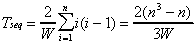. (7.1)

Assuming there are 1 < P n processors. Each processor can eliminate an independent variable within a column. Tcomp is: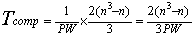.

For each wave i, the size of data volume is 28(n-i)2 (assuming double precision arithmetic), because each processor must take a row in A and return the revised row to update A. We have Tcomm as follows: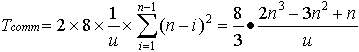.

Further, since the static synchronization is accounted for in the summations of Tcomp and Tcomm, we have Tsync = 0. The parallel timing model is then: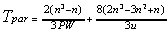. (7.2)

The speedup model is:. (7.3)

The inhibiting factor for this parallel implementation is the O(n3) communication volume. Unless the network speed u >> PW (7.3), the total parallel processor power, the communication overhead offsets all benefits of parallel processing (see the < 100 MBPS lines in Figure 6). Current technological trends indicate that P W can easily exceed u.

Greater efficiency can be brought by using a smaller number of processors and some algorithmic improvements.  shows that it is possible to use a divide-and-conquer strategy (block factorization) to reduce the communication volume to O(n2) with a large constant. This algorithm would then have the similar performance behavior as the matrix multiplication algorithm.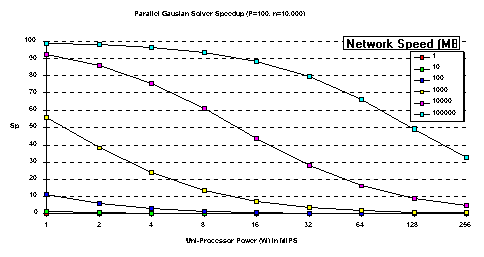Figure 6. Gaussian Solver Speedup Using Different Processors

Synchronous and asynchronous iterative solvers are SSC since their verification and solution algorithms are of the same order. The use of steady state models helps to quantify the frequency of communication in order to obtain the best possible overall processing time .

## 8. Finding the Best Grain Size

Parallel processing grain size affects two major forms of overhead: communication and synchronization. Fine grain parallel processing permits a large number of independent processors at the expense of increased communication overhead. Coarse grain parallel processing saves communication overhead while risking synchronization penalty . Since the computing density of a process is input dependent, the synchronization overhead exists for parallel computers using homogeneous and heterogeneous processors. In the following discussion, we identify the best grain sizes assuming the best load-balancing. In order to achieve the optimal performance, it is necessary to adapt the identified solutions using practical load-balancing algorithms .

Defining the best parallel processing grain used to be an architecture dependent problem. Namely, one must first understand the features of a parallel processor before deciding the "best fit" between the application and these features. It was necessary since older parallel processors typically used low power uni-processors with small local memories.

As low-cost high performance commodity uni-processors become widely available, this "old-fashioned" programming paradigm needs an re-evaluation, especially with the decreasing cost of memory devices. In order to find the true potential of an algorithm, we prefer finding the best possible parallel performance before compromising to architecture specific restrictions. This will give us the "big picture" with marked costs for the compromising details.

For example, a typical Mandelbrot fractal program has three nested loops as follows.

Input:

```
a (magnifying glass real coordinate),

b (magnifying glass complex coordinate),

size (magnifying glass size),

limit (maximal visible colors),

n (display resolution)

```

Program:

```
1. for i=1 to x

2. 	ca = a + i * size/x

3. 	for j=1 to y

4. 		zx = 0

5. 		zy = 0

6. 		count = 0

7. 		cb = b + j * size/y

8. 		while ((zx*zx+zy*zy)<4) and (count < limit)

9. 			count = count + 1

10. 			nzy = 2 * zx * zy + cb

11. 			zx = zx*zx - zy*zy+ ca

12. 			zy = nzy

13. 		end while

14. 		plot at (i,j) with color (count)

15. 	end for

16. end for

```

The sequential and parallel timing models are shown in (4.1) and (4.2).

In search for the largest computing/communication ratio, we study the factors that impact communication overheads since the computing density is determined by the given input.

First, given problem size, for this program the result data volume is a constant. Secondly, the work distribution data volume varies according to partitioning strategies. Assuming single precision arithmetic (4 bytes per number), there are at least three partitioning possibilities with varying communication penalties:

1. Partition the first loop (line 1). This corresponds to "a strip per processor". It requires distributing 4x bytes of data (x times of (i)).
2. Partition the second loop (line 3). This corresponds to "a pixel per processor." It requires distributing 8xy bytes of data (xy times of (i,j)).
3. Partition the statements within the third loop. (lines 9,10,11). This corresponds to three processors per pixel. It requires distributing 20xy bytes of data (xy times of (zx,zy,ca,cb,count)).

Sinceremains the same for a given input and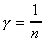of total communication volume (distribution + result data volume), equations (4.3) and (4.4) indicate that cutting the first loop can produce the largestratio (grain size =n/x=y).

Further, if P << x, packing working assignments with xy/P columns each can reduce the data distribution volume to 2 4P (compared to 4x). The optimal grain size is between [xy/P, y], that should be determined experimentally according to some load balancing algorithm.

For Monte Carlo simulators, parallelism does not exist on the top level (t). The speedup model (5.3) indicates that the next highest level (n) should be exploited. (5.3) indicates that P < n is a good choice. Similar to the fractal program, the optimal grain size is between [n/P, n/kP], 1 < k < n/P, adjusted according to some load balancing algorithm.

For parallel sorters, the parallel models (3.2) and (3.5) do not include the switching costs (time for switching from communicating with one processor to another). Intuitively the smallest possible grain size is 1 (implying P=n). In this case, however, both parallel algorithms degenerate into an inefficient insertion sort algorithm (O(n2)). To avoid such degeneration, the only choice left is P < n or grain size = n/P. It is also desirable to have smaller P values since it is part of the parallel overhead ((3.2) and (3.5)).

For parallel matrix multiplication, the intuitive grain level is one dot-product per processor (or P = n2). However, the parallel timing model (6.2) suggests a large communication overhead ((n4)) that would offset all benefits of parallel processing. Similarly, P = n and other finer grain partitioning are equally undesirable. The only choices are P < n or grain size > n2/P.

For a parallel Gaussian Elimination program, parallelism decreases as the calculation progresses. The natural choice is P n or one variable elimination per processor. Coarser grain parallel processes can save on communication costs (7.2). Finer grain parallelism will entail larger communication overhead as illustrated above (case (c)).

We can conclude from all above examples that the highest abstraction level that preserves application specific SIMD, MIMD and pipelined potential is the best parallel processing grain level. Due to hardware implementation difficulties, this coarse grain requirement is not well supported by existing parallel computers. There are many parallel processors that permit high interconnection capacity only at much smaller grain sizes. The timing models can then be used to compare the potential speed difference between the fine and coarse grain designs. The generalization to other algorithms is straightforward.

## 9. Accuracy Control

For studies of a specific parallel processor, modeling accuracy becomes important. Modeling accuracy is affected by both the model precision and parameter accuracy. Model precision depends on the skills of the modeler. Assuming precise models, this section illustrates practical steps toward obtaining accurate values of algorithmic and processing environment parameters. These values define the base points on the performance maps. Curves can then be generated using respective performance models.

### 9.1 Algorithmic Parameters

For most algorithms, there are only three time independent parameters: computing densitycommunication densityand input/output density. Values of these parameters can be obtained by instrumentation statements inserted into the serial program. Since they are time independent, there is no consequence for this "intrusion".

For example, the value of=32,000 in Figure 3 was calculated by counting the total number of operations for plotting the fractal image of complex domain (1,1) (-1,-1) divided by the total number of pixels. Similarlyandcan be obtained.

### 9.2 Processing Environment Parameters

Uni-processor capacity W, interconnect capacity u and input/output capacity B have time dependent values and are generally difficult to obtain. We overcome the difficulties by defining them as "customized measures" for each application. The following steps can ensure a reasonable result.

By definition, B is the average input/output capacity in bytes per second. Inserting counting and timing instructions to the base serial program can get an estimated B value (typically < 10 MBPS for standard non-dedicated SCSI-2 systems, a little higher for dedicated systems without parallel support).

Similarly, W is the application dependent average processing capacity of each processor. Due to the inherent differences between parallel and serial processes solving the same problem, assuming heterogeneous processors, we use the following heuristic to capture its value:

• Record the elapsed times ([ti]) of running the serial program on P uni-processors. The serial program should be solving a problem of n/P size to minimize the resource impact.
• Derive delivered [Wi] using the sequential steady state model, estimated B and [ti].
• The estimated W = [Wi]/P.

For communication capacity (u), we use the following:

• Record the elapsed time (t2) of a parallel run solving the problem of size n using two processors.
• Record the elapsed time (tp) of a parallel run solving the same problem using k>2 processors.
• Use the parallel timing model to derive u2 from B, t2 and W.
• Use the parallel timing model to derive up from B, tp and W.

These are the upper (u2) and lower (up) bounds of u. For SSC algorithms, negative u (communication) values indicate under-estimated W(processor capacity). For NSSC algorithms, we have to find an input instance that would force the parallel system to follow a close-to worst-case search path and re-run the tests.

For most existing multi-processor systems, the input/output capacity B is also dependent on P, since they share the same network. One can then obtain the upper (B2) and lower (Bp) bounds of B using the similar procedure for u.

These values define the base points on the performance maps. Multi-scenario analysis can then be conducted using any values between the upper and lower bounds. If the goal is only to have qualitative conclusions, we can omit detailed statistical analysis. Otherwise, one can use various distributions to approximate the actual behavior of W, u and B. The timing models can then be applied to each time step.

## 10. Conclusions

Steady state timing models are found uses in the following situations:

• For parallel processors employing different inter-connection networks and multiple variously powered uni-processors. The analysis results can influence hardware construction, purchase and program funding decisions.
• For program partition and processing grain definitions in a given parallel processing environment. The analysis result can impact parallel program design and processing efficiency.
• For changing parallel implementation details of a running parallel program, such as increased message sizes, improved hardware messaging support, increased problem size and different input data sets. The analysis results can facilitate the maintenance of parallel programs (and systems).

These assessments can guide effective parallel system development preventing costly mistakes in early stages.

A parallel program has the potential to accelerate its processing speed. However, it also embodies new forms of friction that cannot be well understood using conventional program analysis methods. Unlike sequential programs whose resource use complexities can be analyzed before programming, a parallel program often relied on programming experiments to explain its behavior. The results of experimentation were only of limited value since they contained uncontrolled factors ranging from programming style to hardware features. For exactly the same reasons, parallel programs could not be easily compared.

The steady state timing method simply relates the non-orthogonal parameters in the time domain by introducing resource capacity specifications. It is worth noting that as the speeds of computing, communication and input/output devices improve, the accuracy of steady state models increases.

In this paper, we have used steady state timing models to reveal a few important insights in parallel processing: finding the best processor/network configuration, finding the best grain size and parallel program comparison. These results have important practical implications. For example, since there is an optimal processor/network configuration for each application input, parallel programs should not include direct manipulation of processors since the optimal number of processors will change as the input changes. For parallel compilers, since the best processing grain is found at the top-level abstraction, the new emphasis should be, in addition to existing local optimization schemes, to analyze the top-level data dependencies and gradually "dig" down to finer grains only if the network can sustain the increased traffic.

The use of steady state timing models encourages the discovery of application specific performance features as opposed to architecture specific features. This suggests a discipline in parallel program design and implementation that can be taught in universities. The analysis results can also help the hardware engineers to build more effective parallel architecture that can endure rapid processor and networking technology changes.

We have used timing models to quantify asynchronous linear system solver implementations. The results showed that it is possible to trade the abundance of computing power for limited network bandwidth by systematically relaxing the data exchange requirements in parallel iterative algorithms . We have also found that it is possible to quantify the overhead of fault tolerant techniques for parallel systems such that an optimal compromise of performance and reliability (performanability) can be defined .

In conclusion, we observe that analytical tools of such nature exist in many engineering disciplines and in sequential programming. For parallel programming to be of wide-spread use, we believe such a tool is necessary. The rapid quantitative improvements in uni-processor and inter-connection network speeds fostered the needs for qualitative changes in parallel programming and system design paradigms. This reported merely makes the needs more explicit.

## Acknowledgments

To my colleagues, Drs. Giorgio Ingargiola, James Korsh, Charles Kapps, Arthur Poe, Xiangcuan Ge, Jianming Zhao, Ruijin Qi and to my students, John Dougherty and Kostas Blathras for proofreading earlier drafts of this paper and for applying the timing model method to their work. To CIS750, CIS669 class students for validating the timing model results using the Synergy parallel programming system.